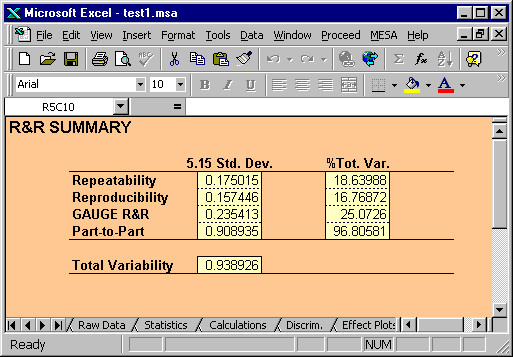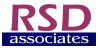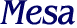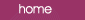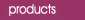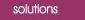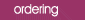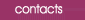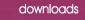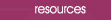R&R Summary The R&R Summary for the AIAG data which is generated by Mesa is shown below: Note that if we add the percentages on the right-hand column they add to well over 100%.  This is a peculiarity of the R&R approach and is caused by the practice of calculating percentages of the standard deviation.  Since standard deviations are not additive then the individual percentages do not add to 100%.  Note that this is one aspect of the R&R approach which has been criticised, and can easily lead to capable measurement systems being labelled as inadequate. Note: When comparing the numerical results in the AIAG manual with those generated by MESA you may find small differences (~1%).  This is because MESA uses the formulae given by Wheeler in his book on industrial experimentation to calculate the values of d2*, rather than take them from an internal table.